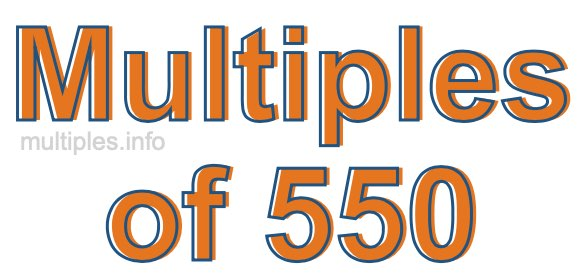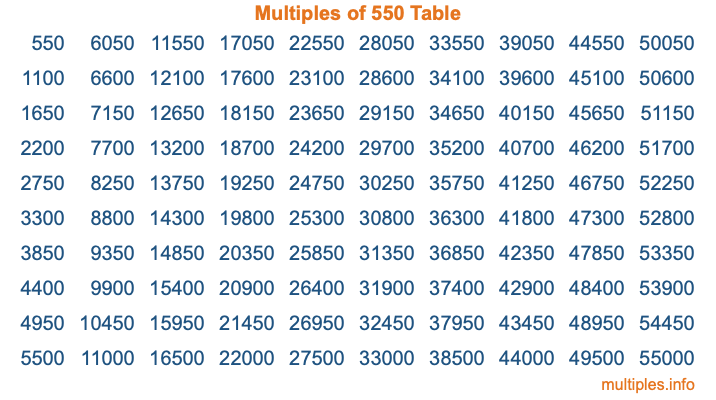Multiples of 550Welcome to the Multiples of 550 page. Here we will first teach you everything you will ever need to know about the multiples of 550, and then give you a study guide summary of everything we taught you to make sure you remember it all. Use this page to look up facts and learn information about the multiples of 550. This page will make you a multiples of five hundred fifty expert!

Definition of Multiples of 550
Multiples of 550 are all the numbers that when divided by 550 equal an integer. Each of the multiples of 550 are called a multiple. A multiple of 550 is created by multiplying 550 by an integer.

Therefore, to create a list of multiples of 550, you start with 1 multiplied by 550, then 2 multiplied by 550, then 3 multiplied by 550, and so on for as long as you want. Thus, the list of the first five multiples of 550 is 550, 1100, 1650, 2200, and 2750. To see a larger list of multiples of 550, see the printable image of Multiples of 550 further down on this page. We also have a category where you can choose any nth multiple of 550.

Multiples of 550 Checker
The Multiples of 550 Checker below checks to see if any number of your choice is a multiple of 550. In other words, it checks to see if there is any number (integer) that when multiplied by 550 will equal your number. To do that, we divide your number by 550. If the the quotient is an integer, then your number is a multiple of 550.

Is  a multiple of 550?

Least Common Multiple of 550 and ...
A Least Common Multiple (LCM) is the lowest multiple that two or more numbers have in common. This is also called the smallest common multiple or lowest common multiple and is useful to know when you are adding our subtracting fractions. Enter one or more numbers below (550 is already entered) to find the LCM.

Check out our LCM Calculator if you need more details about the Least Common Multiple or if you need the LCM for different numbers for adding and subtraction fractions.

nth Multiple of 550
As we stated above, 550 is the first multiple of 550, 1100 is the second multiple of 550, 1650 is the third multiple of 550, and so on. Enter a number below to find the nth multiple of 550.

th multiple of 550

Multiples of 550 vs Factors of 550
550 is a multiple of 550 and a factor of 550, but that is where the similarities end. All postive multiples of 550 are 550 or greater than 550. All positive factors of 550 are 550 or less than 550.

Below is the beginning list of multiples of 550 and the factors of 550 so you can compare:

Multiples of 550: 550, 1100, 1650, 2200, 2750, etc.

Factors of 550: 1, 2, 5, 10, 11, 22, 25, 50, 55, 110, 275, 550

As you can see, the multiples of 550 are all the numbers that you can divide by 550 to get a whole number. The factors of 550, on the other hand, are all the whole numbers that you can multiply by another whole number to get 550.

It's also interesting to note that if a number (x) is a factor of 550, then 550 will also be a multiple of that number (x).

Multiples of 550 vs Divisors of 550
The divisors of 550 are all the integers that 550 can be divided by evenly. Below is a list of the divisors of 550.

Divisors of 550: 1, 2, 5, 10, 11, 22, 25, 50, 55, 110, 275, 550

The interesting thing to note here is that if you take any multiple of 550 and divide it by a divisor of 550, you will see that the quotient is an integer.

Multiples of 550 Table
Below is an image of the first 100 multiples of 550 in a table. The table is in chronological order, column by column. The first column has the first ten multiples of 550, the second column has the next ten multiples of 550, and so on.The Multiples of 550 Table is also referred to as the 550 Times Table or Times Table of 550. You are welcome to print out our table for your studies.

Negative Multiples of 550
Although not often discussed or needed in math, it is worth mentioning that you can make a list of negative multiples of 550 by multiplying 550 by -1, then by -2, then by -3, and so on, to get the following list of negative multiples of 550:

-550, -1100, -1650, -2200, -2750, etc.

Multiples of 550 Summary
Below is a summary of important Multiples of 550 facts that we have discussed on this page. To retain the knowledge on this page, we recommend that you read through the summary and explain to yourself or a study partner why they hold true.

There are an infinite number of multiples of 550.

A multiple of 550 divided by 550 will equal a whole number.

550 divided by a factor of 550 equals a divisor of 550.

The nth multiple of 550 is n times 550.

The largest factor of 550 is equal to the first positive multiple of 550.

550 is a multiple of every factor of 550.

550 is a multiple of 550.

A multiple of 550 divided by a divisor of 550 equals an integer.

550 divided by a divisor of 550 equals a factor of 550.

Any integer times 550 will equal a multiple of 550.

Multiples of a Number
Here you can get the multiples of another number, all with the same attention to detail as we did for multiples of 550 on this page.

Multiples of
Multiples of 551
Did you find our page about multiples of five hundred fifty educational? Do you want more knowledge? Check out the multiples of the next number on our list!